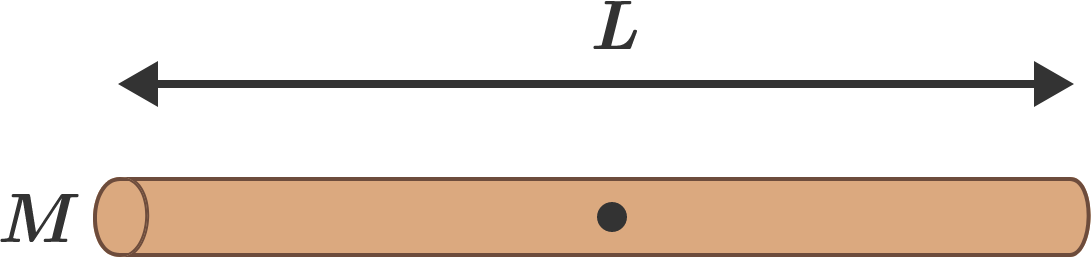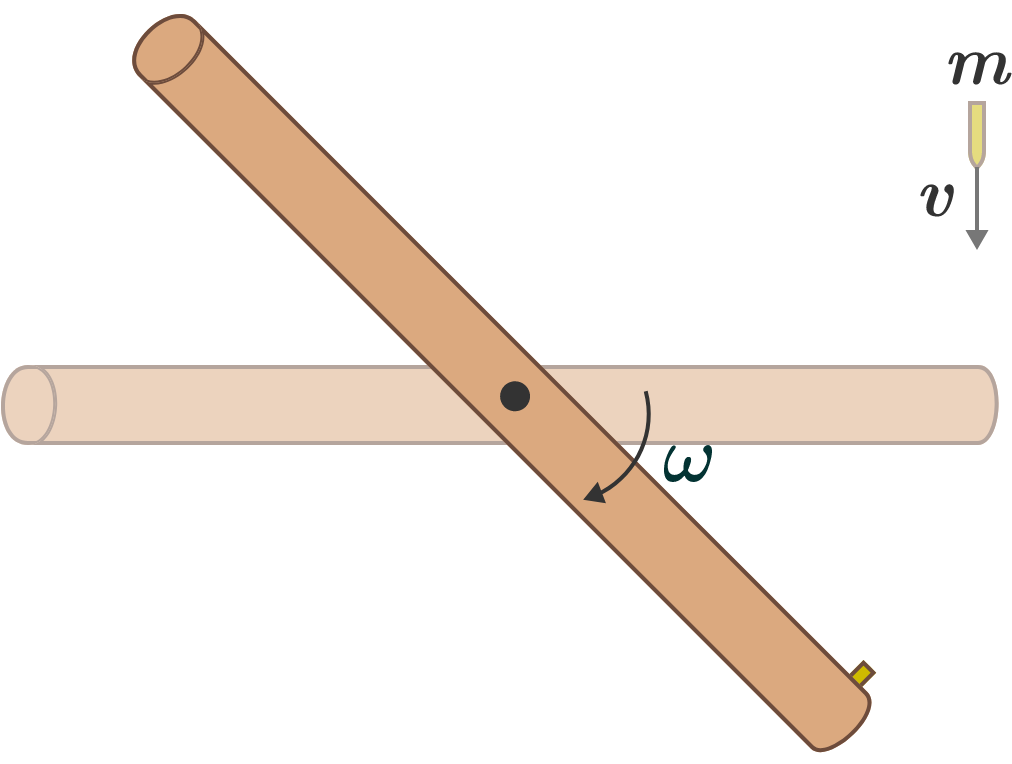# An analysis of a rotating rod

Consider a uniform rod of mass $M$ and length $L,$ free to rotate around a frictionless axis passing through its center and going into the page. Initially, the rod is stationary in the horizontal position, as shown in the diagram below.Now, a small bullet of mass $m$ moving with velocity $v$ hits the rod at its extreme end and sticks to it. The system rotates vertically through some angle $\theta$ before it momentarily comes to rest. If this angle can be expressed (in degrees) as $\theta = \alpha + \arcsin \left(\frac {\beta mv^2}{( M+\gamma m)gL}\right),$ where $g$ denotes the gravitational acceleration and $\alpha$, $\beta$, and $\gamma$ are positive integer constants with $\alpha$ in degrees, then find the value of $\alpha + \beta + \gamma$.×